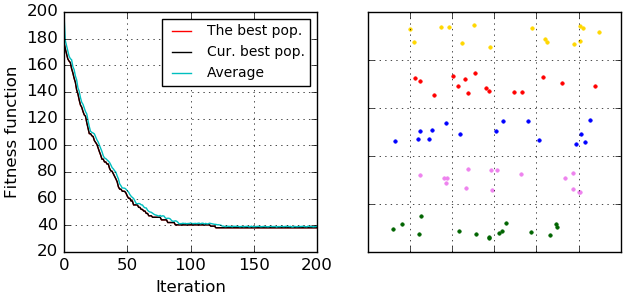pyclustering.cluster.ga.genetic_algorithm Class Reference

Class represents Genetic clustering algorithm. More...

## Public Member Functions

def __init__ (self, data, count_clusters, chromosome_count, population_count, kwargs)
Initialize genetic clustering algorithm for cluster analysis. More...

def process (self)
Perform clustering procedure in line with rule of genetic clustering algorithm. More...

def get_observer (self)
Returns genetic algorithm observer.

def get_clusters (self)
Returns list of allocated clusters, each cluster contains indexes of objects from the data. More...

## Detailed Description

Class represents Genetic clustering algorithm.

The searching capability of genetic algorithms is exploited in order to search for appropriate cluster centres.

Example of clustering using genetic algorithm:

from pyclustering.cluster.ga import genetic_algorithm, ga_observer
from pyclustering.samples.definitions import SIMPLE_SAMPLES
# Read input data for clustering
# Create instance of observer that will collect all information:
observer_instance = ga_observer(True, True, True)
# Create genetic algorithm for clustering
ga_instance = genetic_algorithm(data=sample,
count_clusters=4,
chromosome_count=100,
population_count=200,
count_mutation_gens=1)
# Start processing
ga_instance.process()
# Obtain results
clusters = ga_instance.get_clusters()
# Print cluster to console
print("Amount of clusters: '%d'. Clusters: '%s'" % (len(clusters), clusters))

There is an example of clustering results (fitness function evolution and allocated clusters) that were visualized by 'ga_visualizer':ga_visualizer
ga_observer

Definition at line 325 of file ga.py.

## ◆ __init__()

 def pyclustering.cluster.ga.genetic_algorithm.__init__ ( self, data, count_clusters, chromosome_count, population_count, kwargs )

Initialize genetic clustering algorithm for cluster analysis.

Parameters
 [in] data (numpy.array|list): Input data for clustering that is represented by two dimensional array where each row is a point, for example, [[0.0, 2.1], [0.1, 2.0], [-0.2, 2.4]]. [in] count_clusters (uint): Amount of clusters that should be allocated in the data. [in] chromosome_count (uint): Amount of chromosomes in each population. [in] population_count (uint): Amount of populations. [in] **kwargs Arbitrary keyword arguments (available arguments: 'count_mutation_gens', 'coeff_mutation_count', 'select_coeff', 'crossover_rate', 'observer').

Keyword Args:

• count_mutation_gens (uint): Amount of genes in chromosome that is mutated on each step.
• coeff_mutation_count (float): Percent of chromosomes for mutation, distributed in range (0, 1] and thus amount of chromosomes is defined as follows: 'chromosome_count' * 'coeff_mutation_count'.
• select_coeff (float): Exponential coefficient for selection procedure that is used as follows: math.exp(1 + fitness(chromosome) * select_coeff).
• crossover_rate (float): Crossover rate.
• observer (ga_observer): Observer that is used for collecting information of about clustering process on each step.

Definition at line 371 of file ga.py.

## ◆ get_clusters()

 def pyclustering.cluster.ga.genetic_algorithm.get_clusters ( self )

Returns list of allocated clusters, each cluster contains indexes of objects from the data.

Returns
(list) List of allocated clusters.
process()

Definition at line 493 of file ga.py.

## ◆ process()

 def pyclustering.cluster.ga.genetic_algorithm.process ( self )

Perform clustering procedure in line with rule of genetic clustering algorithm.